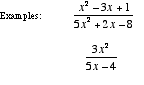index: click on a letter A B C D E F G H I J K L M N O P Q R S T U V W X Y Z A to Z index index: subject areas numbers & symbols sets, logic, proofs geometry algebra trigonometry advanced algebra & pre-calculus calculus advanced topics probability & statistics real world applications multimedia entrieswww.mathwords.com about mathwords website feedback

 Improper Rational Expression A rational expression in which the degree of the numerator polynomial is greater than or equal to the degree of the denominator polynomial. Note: Polynomial long division can be used to write an improper rational expression as the sum of a polynomial and a proper rational expression. Synthetic division may also be used for some fractions.See also